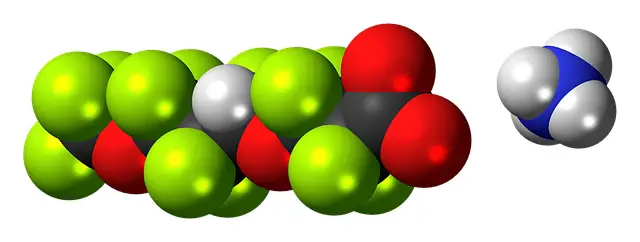# Any Difference between mole and molecule? |Any difference between mol or mole?

Last updated on April 16th, 2021 at 12:53 pm

While studying the chapter on gas laws you must have come across the term ‘mole’ or ‘mol‘.
You may have seen gram-mol as well in that chapter.
I find many students confused with mole (or mol) and molecule.
Here we will discuss and explain the concept of mole or mol with some examples.
1> In the beginning, we will see if mol and mole are different or the same. (in section difference between mole and mole – if any)
2> Then we’ll also go through a quick definition of molecules before discussing mole vs molecule. This will help you to understand the difference between mole and molecule.

## What is the difference between mol and mole?

Any difference between mol or mole? The answer is “No”. Note that mole and mol are synonymous. (same thing).

## what is Mole or Mol?

One mole (also abbreviated as mol) is the amount of substance that contains a specific number of particles ( 6.023 X 1023). These particles may be atoms or molecules or ions etc. Just to simplify, 1 mole of sand means a sand sample where 6.023 X 1023 number of sand particles are there.
So if we say 1 mol of Oxygen gas, then that means an amount of Oxygen sample which contains 6.023 X 1023 number of Oxygen molecules. (in gas form of Oxygen is available in molecules)
Similarly, 1 mol of atomic Carbon contains 6.023 X 1023 number of Carbon atoms. (in atomic form of Carbon, it is available in atoms)
This specific number (6.023 X 1023 ) is called the Avogadro number.

See also  km/h to m/s - unit conversion from kilometer/hour to meter/second

## What is the difference between mole and molecule?

molecule is the smallest particle in an element or compound that possesses the chemical properties of that element or compound. Molecules are made up of atoms that are again held together by chemical bonds. But, a Mol or Mole is an amount of substance and it may contain particles of atoms or molecules or ions, etc. of a certain specific number. This specific number (6.023 X 1023 ) is called the Avogadro number.

## What is Gram-mole? What is the representation of molar mass in gram?

### What do you mean by 1 gram mole of Oxygen gas?

Oxygen gas exists in molecular form in nature.

So, in this case, we have to count the molecular mass of Oxygen( O = 2 x 16) and add the gram unit with it to get the mass of 1 gram mole Oxygen.

So 1 gram mole Oxygen gas means 32 grams of Oxygen gas.

### What do you mean by 1 gram mole atomic Carbon?

Carbon is available in the atomic form.

So, in this case, we have to get the atomic mass of Carbon (C = 1X12) and again add the gram unit with it.

So 1 gram mole atomic Carbon means 12 gram Carbon.

### What do you mean by 1 gram mole water (H2O)?

As water (steam/vapor) is available in molecular form, so 1 gram mole water (H2O) means 2 x 1 + 16 that is 18 grams of water molecules.

## What is the relationship between Gram mole and Avogadro number?

So we can say that 32 grams of Oxygen gas will contain Avogadro number (6.023 X 1023) of Oxygen molecules.

Similarly, 12 grams of atomic Carbon contains Avogadro number (6.023 X 1023) of Carbon atoms.

And 18 grams of water molecules will contain Avogadro number (6.023 X 1023) of water molecules.

**Remember that (6.023 X 1023 ) is called Avogadro number.

## Sample problems:

Q1. Find out the number of moles in 132 grams of CO2.

Solution:

Molar mass of CO2 = gram molecular mass of CO2 as it is available in molecular form = [12 + 2X16] gram = 44 gram.

we know, the total mass of the sample = mass per mole X number of moles = molar mass X number of moles

so, number of moles (n) = mass of the sample/mass per mole = 132/44 =3

Q2. Find out the number of molecules in 8 grams of Oxygen.

Solution: you can try and do it yourself. (Ans: 1.506 X 10^23 molecules)

Suggested Post: physics problems

Scroll to top
error: physicsTeacher.in